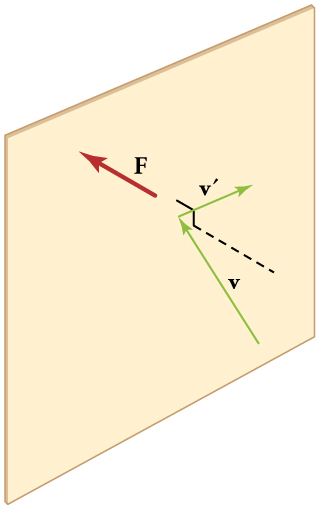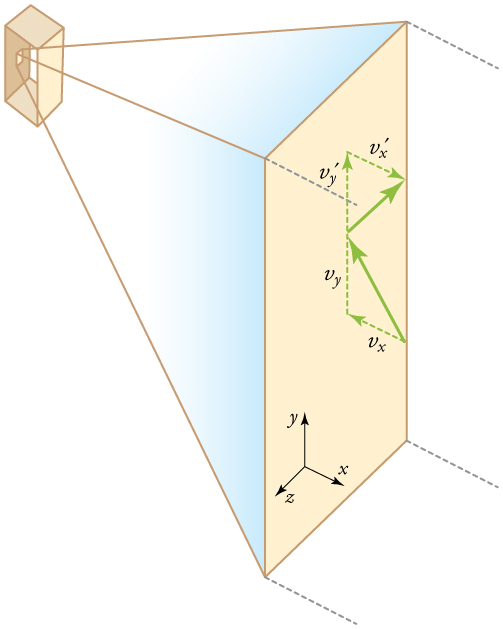# 13.4 Kinetic theory: atomic and molecular explanation of pressure

 Page 1 / 5
• Express the ideal gas law in terms of molecular mass and velocity.
• Define thermal energy.
• Calculate the kinetic energy of a gas molecule, given its temperature.
• Describe the relationship between the temperature of a gas and the kinetic energy of atoms and molecules.
• Describe the distribution of speeds of molecules in a gas.

We have developed macroscopic definitions of pressure and temperature. Pressure is the force divided by the area on which the force is exerted, and temperature is measured with a thermometer. We gain a better understanding of pressure and temperature from the kinetic theory of gases, which assumes that atoms and molecules are in continuous random motion.When a molecule collides with a rigid wall, the component of its momentum perpendicular to the wall is reversed. A force is thus exerted on the wall, creating pressure.

[link] shows an elastic collision of a gas molecule with the wall of a container, so that it exerts a force on the wall (by Newton’s third law). Because a huge number of molecules will collide with the wall in a short time, we observe an average force per unit area. These collisions are the source of pressure in a gas. As the number of molecules increases, the number of collisions and thus the pressure increase. Similarly, the gas pressure is higher if the average velocity of molecules is higher. The actual relationship is derived in the Things Great and Small feature below. The following relationship is found:

$\text{PV}=\frac{1}{3}\text{Nm}\overline{{v}^{2}},$

where $P$ is the pressure (average force per unit area), $V$ is the volume of gas in the container, $N$ is the number of molecules in the container, $m$ is the mass of a molecule, and $\overline{{v}^{2}}$ is the average of the molecular speed squared.

What can we learn from this atomic and molecular version of the ideal gas law? We can derive a relationship between temperature and the average translational kinetic energy of molecules in a gas. Recall the previous expression of the ideal gas law:

$\text{PV}=\text{NkT}.$

Equating the right-hand side of this equation with the right-hand side of $\text{PV}=\frac{1}{3}\text{Nm}\overline{{v}^{2}}$ gives

$\frac{1}{3}\text{Nm}\overline{{v}^{2}}=\text{NkT}.$

## Making connections: things great and small—atomic and molecular origin of pressure in a gas

[link] shows a box filled with a gas. We know from our previous discussions that putting more gas into the box produces greater pressure, and that increasing the temperature of the gas also produces a greater pressure. But why should increasing the temperature of the gas increase the pressure in the box? A look at the atomic and molecular scale gives us some answers, and an alternative expression for the ideal gas law.

The figure shows an expanded view of an elastic collision of a gas molecule with the wall of a container. Calculating the average force exerted by such molecules will lead us to the ideal gas law, and to the connection between temperature and molecular kinetic energy. We assume that a molecule is small compared with the separation of molecules in the gas, and that its interaction with other molecules can be ignored. We also assume the wall is rigid and that the molecule’s direction changes, but that its speed remains constant (and hence its kinetic energy and the magnitude of its momentum remain constant as well). This assumption is not always valid, but the same result is obtained with a more detailed description of the molecule’s exchange of energy and momentum with the wall.Gas in a box exerts an outward pressure on its walls. A molecule colliding with a rigid wall has the direction of its velocity and momentum in the x size 12{x} {} -direction reversed. This direction is perpendicular to the wall. The components of its velocity momentum in the y size 12{y} {} - and z size 12{z} {} -directions are not changed, which means there is no force parallel to the wall.

If the molecule’s velocity changes in the $x$ -direction, its momentum changes from $–{\text{mv}}_{x}$ to $+{\text{mv}}_{x}$ . Thus, its change in momentum is $\text{Δ}\text{mv}\phantom{\rule{0.20em}{0ex}}\text{= +}{\text{mv}}_{x}–\left(–{\text{mv}}_{x}\right)=2{\text{mv}}_{x}$ . The force exerted on the molecule is given by

$F=\frac{\text{Δ}p}{\text{Δ}t}=\frac{2{\text{mv}}_{x}}{\text{Δ}t}\text{.}$

There is no force between the wall and the molecule until the molecule hits the wall. During the short time of the collision, the force between the molecule and wall is relatively large. We are looking for an average force; we take $\text{Δ}t$ to be the average time between collisions of the molecule with this wall. It is the time it would take the molecule to go across the box and back (a distance $2l\right)$ at a speed of ${v}_{x}$ . Thus $\text{Δ}t=2l/{v}_{x}$ , and the expression for the force becomes

$F=\frac{2{\text{mv}}_{x}}{2l/{v}_{x}}=\frac{{\text{mv}}_{x}^{2}}{l}\text{.}$

This force is due to one molecule. We multiply by the number of molecules $N$ and use their average squared velocity to find the force

$F=N\frac{m\overline{{v}_{x}^{2}}}{l},$

where the bar over a quantity means its average value. We would like to have the force in terms of the speed $v$ , rather than the $x$ -component of the velocity. We note that the total velocity squared is the sum of the squares of its components, so that

$\overline{{v}^{2}}=\overline{{v}_{x}^{2}}+\overline{{v}_{y}^{2}}+\overline{{v}_{z}^{2}}\text{.}$

Because the velocities are random, their average components in all directions are the same:

$\overline{{v}_{x}^{2}}=\overline{{v}_{y}^{2}}=\overline{{v}_{z}^{2}}\text{.}$

Thus,

$\overline{{v}^{2}}=3\overline{{v}_{x}^{2}},$

or

$\overline{{v}_{x}^{2}}=\frac{1}{3}\overline{{v}^{2}}.$

Substituting $\frac{1}{3}\overline{{v}^{2}}$ into the expression for $F$ gives

$F=N\frac{m\overline{{v}^{2}}}{3l}\text{.}$

The pressure is $F/A,$ so that we obtain

$P=\frac{F}{A}=N\frac{m\overline{{v}^{2}}}{3\text{Al}}=\frac{1}{3}\frac{\text{Nm}\overline{{v}^{2}}}{V},$

where we used $V=\text{Al}$ for the volume. This gives the important result.

$\text{PV}=\frac{1}{3}\text{Nm}\overline{{v}^{2}}$

This equation is another expression of the ideal gas law.

write an expression for a plane progressive wave moving from left to right along x axis and having amplitude 0.02m, frequency of 650Hz and speed if 680ms-¹
how does a model differ from a theory
what is vector quantity
Vector quality have both direction and magnitude, such as Force, displacement, acceleration and etc.
Besmellah
Is the force attractive or repulsive between the hot and neutral lines hung from power poles? Why?
what's electromagnetic induction
electromagnetic induction is a process in which conductor is put in a particular position and magnetic field keeps varying.
Lukman
wow great
Salaudeen
what is mutual induction?
je
mutual induction can be define as the current flowing in one coil that induces a voltage in an adjacent coil.
Johnson
how to undergo polarization
show that a particle moving under the influence of an attractive force mu/y³ towards the axis x. show that if it be projected from the point (0,k) with the component velocities U and V parallel to the axis of x and y, it will not strike the axis of x unless u>v²k² and distance uk²/√u-vk as origin
show that a particle moving under the influence of an attractive force mu/y^3 towards the axis x. show that if it be projected from the point (0,k) with the component velocities U and V parallel to the axis of x and y, it will not strike the axis of x unless u>v^2k^2 and distance uk^2/√u-k as origin
No idea.... Are you even sure this question exist?
Mavis
I can't even understand the question
yes it was an assignment question "^"represent raise to power pls
Gabriel
Gabriel
An engineer builds two simple pendula. Both are suspended from small wires secured to the ceiling of a room. Each pendulum hovers 2 cm above the floor. Pendulum 1 has a bob with a mass of 10kg . Pendulum 2 has a bob with a mass of 100 kg . Describe how the motion of the pendula will differ if the bobs are both displaced by 12º .
no ideas
Augstine
if u at an angle of 12 degrees their period will be same so as their velocity, that means they both move simultaneously since both both hovers at same length meaning they have the same length
Modern cars are made of materials that make them collapsible upon collision. Explain using physics concept (Force and impulse), how these car designs help with the safety of passengers.
calculate the force due to surface tension required to support a column liquid in a capillary tube 5mm. If the capillary tube is dipped into a beaker of water
find the time required for a train Half a Kilometre long to cross a bridge almost kilometre long racing at 100km/h
method of polarization
Ajayi
What is atomic number?
The number of protons in the nucleus of an atom
Deborah
type of thermodynamics
oxygen gas contained in a ccylinder of volume has a temp of 300k and pressure 2.5×10Nm
why the satellite does not drop to the earth explain
what is a matter
Yinka
what is matter
Yinka
what is matter
Yinka
what is a matter
Yinka
I want the nuclear physics conversation
Mohamed
because space is a vacuum and anything outside the earth 🌎 can not come back without an act of force applied to it to leave the vacuum and fall down to the earth with a maximum force length of 30kcm per second
Clara

#### Get Jobilize Job Search Mobile App in your pocket Now!ByBy OpenStaxBy OpenStaxBy OpenStaxBy Abishek DevarajBy Maureen MillerBy Hope PercleBy Alec MoffitBy OpenStaxByBy OpenStax